Inductive Reactance and Capacitive Reactance

In this lesson, we will explore the concepts of inductive reactance and capacitive reactance and try to understand all the underlying terms. Inductive reactance is usually related to the magnetic field surrounding a wire or a coil carrying current. Likewise, capacitive reactance is often linked with the electric field that keeps changing between two conducting plates or surfaces that are kept apart from each other by some insulating medium.

However, before we learn about these topics in detail we will first recall the definition of reactance and then move on to understand its types. Inductive reactance and capacitive reactance are the two main types of reactance.

What is Reactance?

Reactance is the opposition offered by capacitor and inductor in a circuit to the flow of AC current in the circuit. It is quite similar to resistance but reactance varies with the frequency of the ac voltage source. It is measured in ohms.

If we look at conductors that are carrying alternating current then we will find that reactance is always present along with resistance. Moreover, reactance also appears in shorter intervals as the direct current changes while approaching or departing from a steady flow.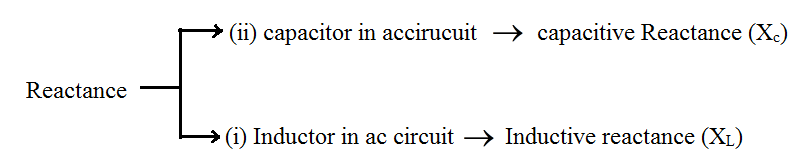We will discuss them in detail below.

Inductive Reactance (XL)

Definition: Inductive reactance is the opposition offered by the inductor in an AC circuit to the flow of ac current.

It is represented by (XL) and measured in ohms (Ω). Inductive reactance is mostly low for lower frequencies and high for higher frequencies. It is, however, negligible for steady DC current.

The inductive reactance formula is given as follows;

Inductive Reactance, XL = 2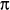fL

The AC circuit with a pure inductor is represented as;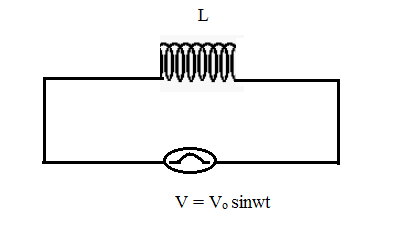By KVL

${{V}_{o}}\sin wt-\frac{Ldi}{dt}=0$ $\int\limits_{0}^{t}{\frac{{{V}_{o}}}{L}}\sin wt\,dt\,=\,\int\limits_{0}^{1}{di}$ $-\frac{{{V}_{o}}}{Lw}\cos wt=i$ $-{{i}_{o}}\cos wt=i$

and

$i_{o}^{{}}=\frac{{{V}_{o}}}{Lw}=\frac{{{V}_{o}}}{{{X}_{L}}}$

XL = Lw = 2πLf (inductive reactance)

XL α L

XL α w 🡪 1

Where

L – is inductance of the coil

W – is angular frequency of AC voltage source.

From equation – 1

W 🡪 Higher frequency 🡪 Higher the resistance to the current flow

High (fhigh) (or)

Current changes more rapidly for higher frequencies

W = 0 🡪 f = 0 🡪 XL = 0

XL (Inductive reactance)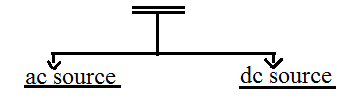w ≠ 0 w = 0

XL = Lw XL = 0

for AC with fhigh 🡪 XL = High for DC XL 🡺 is zero

for AC with flow 🡪 XL = small XL = resistance of the wire in a circuit.

Since from phasor diagram for AC circuit with an only resistor, capacitor and inductor.

For AC circuit with only a resistor and its phasor diagram:

AC circuit with only a resistor shows that current and voltage are in the same phase. Which means an increase in voltage leads to an increase in current (vice – versa)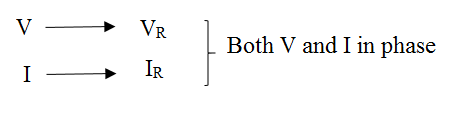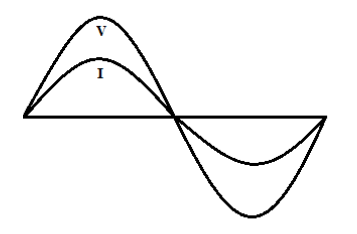For AC circuit with an only inductor (L);

Since we know that V = Vo sinwt

i = io coswt = io sin $\left( wt=\frac{-\pi }{2} \right)$

Current lags behind the voltage with phase difference $\frac{\pi }{2}$ between them.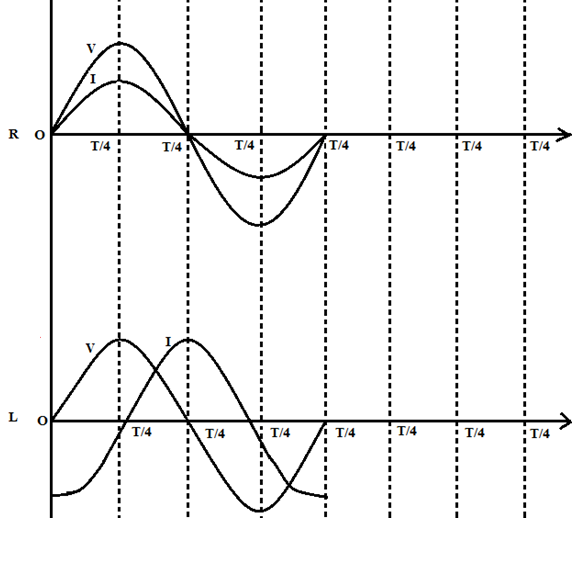AC circuit with R only

V = vo sinwt

i = io sinwt

AC circuit with L

V = vo sinwt

i = io sinwt

(i) As we can clearly see from the given phasor diagram above, in an AC circuit with a pure resistor the AC current through it and the AC voltage across it rises together (or) falls together which indicates both current and voltage are in phase.

(ii) But in the case of AC circuit with L(only), it is clearly seen from the given phasor diagram V and I are in out of phase. Which means if V = max then i = minimum.

(iii) This is because of the characteristics of the inductor. Inductance always opposes a change in current which means that current through an inductor continuously reverses itself.

(iv) Lenz’s Law:

According to Lenz’s Law, a circuit with inductance offers opposing force to the change in current inducing counter emf (induced emf) maintaining the phase between v and I which remains the same.

(v) We can see the above fig as four cycles.

(a) First (T/4) cycle:

Applied Vo Hage starts increasing from zero.

When v = o, i = – Ve (max) = -imax

When v = Vmax,i = 0

(b) Second (T/4) cycle:

Applied voltage is starts decreasing from Vmax.

When V reaches:

V = o i = imax

(c) Third (T/4) cycle:

The applied voltage starts increasing in the opposite direction (or) reverse direction.

When V reaches, V = – Vmax,

i starts decreasing reaches i = 0

(d) Fourth (T/4) cycle:

The applied voltage starts decreasing from – Vmax to zero but i reaches the –imax.

V = 0 , i = – imax

Inertia Effect By Inductor:

We can see from the above analysis V and i are continuously changing in magnitude and direction this is because of the inductor continuously reverses the current through it by itself due to the inertia effect of emf.

Inertia effect for > inertia effect for DC

Similarly for AC,

Greater the value of (L) inductance 🡪 greater the opposition by inertia effect

Faster the reversal of current 🡪 greater the opposition by inertia effect.

The opposition that is offered by inductor to the flow of an alternating current we cannot call it as resistance because this not friction within the conductor, this is the reaction of the inductor to the change in alternating current.

Special Cases

An inductor (L) 🡪 have appreciable XL for AC source and reduces the amount of current.

Case (I): Inductor and a Bulb in series with an AC source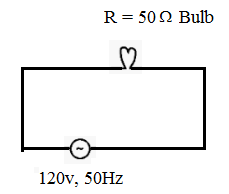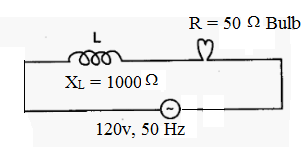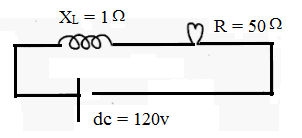(i) circuit without inductor (ii) circuit with High inductor (iii) circuit with Low inductor

There is no inductor, XL = 1000Ω for DC inductor offers

AC source causes the Bulb glows $i=\frac{V}{{{X}_{L}}}=\frac{120}{1000}$ XL = 0

with full Bright = 0.012 A i = 1.4 A

$i=\frac{V}{R}=\frac{120}{50}=1.4A$ Here the glows with full bright

Case (II): Combination of Inductive Reactance

XL = provides opposition to an AC source. So it summed in the same way as resistance.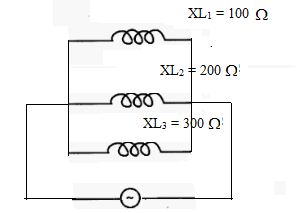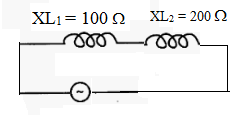XLeft = XL1 + XL2 $\frac{1}{{{X}_{Left}}}=\frac{1}{{{X}_{L}}_{_{1}}}+\frac{1}{{{X}_{L}}_{_{2}}}+\frac{1}{{{X}_{L}}_{_{3}}}$

Case (III): Current in AC circuit with inductors only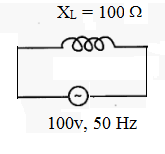. $i=\frac{v}{X_L} = 1A$ .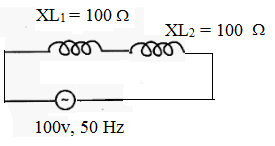$V = v_1+V_2$

= I × L1+IXL2

= I(X L1+XL2)

= $I = \frac{V}{XL_1+XL_2} = \frac{V}{X_{left}}$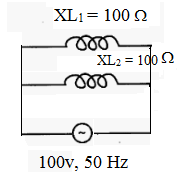${{I}_{1}}=\frac{V}{{{X}_{{{L}_{1}}}}}=1A$ ${{I}_{2}}=\frac{V}{{{X}_{L}}_{_{2}}}=1A$ $I={{I}_{1}}+{{I}_{2}}=2A$.

I = 1/2 A

Application Of Inductive Reactance

XL – for AC offers minimum reactance to AC at low frequency and maximum reactance to AC at high frequency.

For DC – XL = 0

Solved Problems

Problem 1: Find out the XL for an AC source of 100v and 50 Hz by an inductor L = 50 mπ?

XL = Lw = 50 × 10–3 × 2π × 50 = 5000π × 10–3 = 5π

Problem: 2 An inductor offers resistance of 100 Ω to an AC source of 100v and 50 Hz. Find out the value of inductor?

XL = LW

100 = L2π 0×50 🡺 L = 1/π

What is the difference between Resistance and Reactance?

Resistance 🡪 is frequency-independent

Reactance 🡪 is frequency-dependent.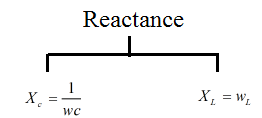1. An inductor is connected to an AC source of 100 v and 50 Hr, what happens to the current in the circuit if the frequency increases?

XL = Lw, for increase in(f) cause increase in XL

$i=\frac{v}{{{X}_{L}}},$ so current will decreases

1. An inductor is connected to an AC source of 100 V and 50 Hr what happens to the current in the circuit if its frequency decreases?

XL = Lw, for decreases in (f) causes decrease in XL

$i=\frac{v}{{{X}_{L}}},$so i will increase.

Capacitive Reactance

What is capacitive resistance? The definition of capacitive reactance states that it is the opposition offered by a capacitor to the flow of ac current in the ac circuit. A capacitor opposes the changes in the potential difference or the voltage across its plates. Capacitive reactance is said to be inversely proportional to the capacitance and the signal frequency. It is normally represented by (Xc) and measured in the SI unit of ohm (Ω).

The capacitive reactance formula is given as follows;

Capacitive Reactance, Xc =  1/2fC

The AC circuit with pure capacitor is represented as;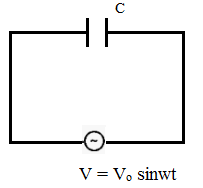According KVL,

${{V}_{o}}\,\sin wt-\frac{q}{c}=$

CVo sinwt = q

$\frac{d}{dt}\left( C{{V}_{o}}\sin wt \right)=\frac{dq}{dt}$

wcvo costwt = i

where wcVo = io

$\frac{{{V}_{o}}}{\frac{1}{wc}}=\frac{{{V}_{o}}}{{{X}_{L}}}={{i}_{o}}$ ${{X}_{c}}=\frac{1}{wc}=capacitive\,reac\tan ce$ ${{X}_{c}}\alpha \frac{1}{w}(or){{x}_{c}}\alpha \frac{1}{f}\,and$ ${{X}_{c}}\alpha \frac{1}{c}$ ${{X}_{c}}=\frac{1}{wc}=\frac{1}{2\pi fc}$

AC current flows through the capacitor when AC voltage applied.

If c – is small 🡺allows small the current

F – is small 🡺 smaller the current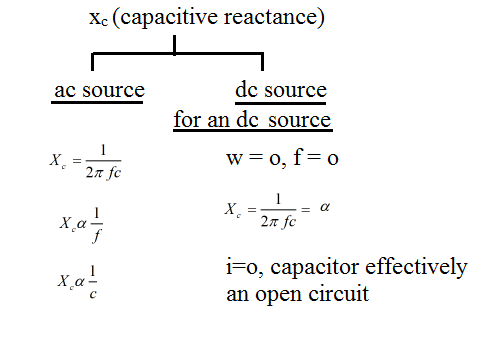Since,

V = Vo sinwt

i = io coswt = io sin $\sin \left( wt+\frac{\pi }{2} \right)$

Current (i) leads with the phase difference π/2 to the applied voltage.

As we know, there is no current through the dielectrics of the capacitor but alternative charging / discharging of the capacitor appears as the current passing through the capacitor.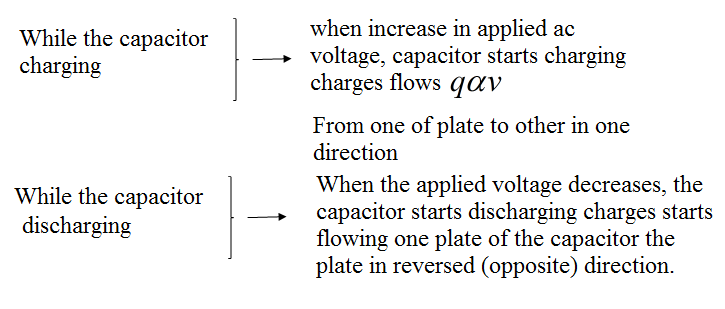When an AC voltage applied 🡪 Alternative charging and discharging happens to the capacitor continuously and it appears as the current passing through the capacitor.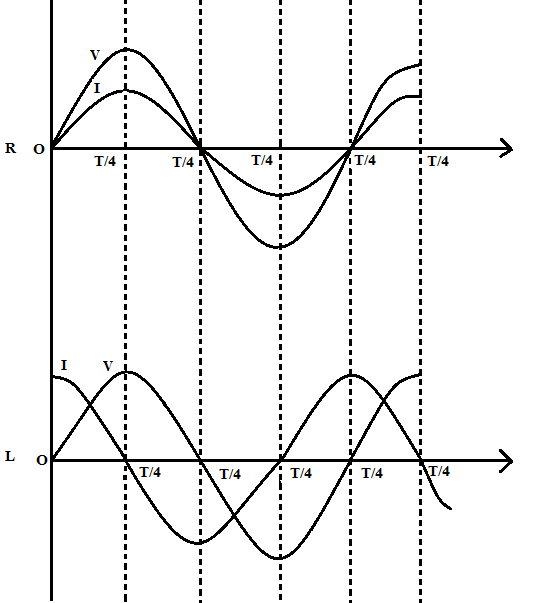AC circuit with a pure resistor

V = vo sinwt

i = io sinwt

AC circuit with a pure capacitor

v = vo sinwt

I – io coswt

The figure above shows the phasor diagram of the AC circuit with a pure resistor and pure capacitor. We can clearly see the difference between the phasor diagram of a pure resistor and pure inductor.

Now will discuss the phasor diagram of the pure capacitor:

From t = 0 to t = T/4, an AC voltage is increasing in positive, change in the AC voltage at its greatest rate as we know that, charge on the capacitor varies directly proportional to the applied voltage. So, it tells that the charge on the capacitor is also changing at its greatest rate. Another words the greater number of electrons are moving off from one plate to another plate.

When V = Vmax, I = o which means the capacitor reached it’s steady (fully charged)

When voltage approaches maximum, change in ac voltage approaches to zero (becomes less), the current must decreases to zero.

Second cycle of (T/4):

V = Vmax to V = approaches to zero.

V is decreasing but still in positive, it means the plates which has accumulated with an excess amount of electron, must lose some electron, the current flow must reverse its direction of the current which is increasing in –ve.

The third cycle of (T/4):

Voltage from, Vo to V = – Vmax

Applied AC voltage polarity is reversed it starts increasing to Vmax in –ve direction when v reaches Vmax which means charges are equally distributed between the plates of the capacitor. At Vmax – capacitor reaches steady state (fully charged) so i reaches i = 0 (zero)

Similarly, the fourth cycle of (T/4):

Again voltage starts decreasing from Vc = Vmax to V = Vo, the capacitor must lose some charges from its negative plates by discharging.

The above discussed four cycles repeat further and further.

Done to the charging / discharging process:

The amplitude and polarity of the AC voltage and amplitude and direction of AC current are continuously charging.

The direction and strength of the current are in relation to the polarity and strength of voltage that appears across the plates of the capacitor which is changing the direction of charges flow at the same rate as AC applied voltage. This makes the capacitor to pass an AC current. Actually, charges do not pass through the dielectric but the rushing effect involving back and forth motion of charges from plate to plate causes the opposition to arise during the flow of charge.

That opposition is (Xc).

For a given AC voltage with a frequency number of charges which can go back and forth between plates of the capacitor is limited by the storage capacity of capacitor which is called capacitance of the capacitor the opposition offered by the capacitor is called capacitive reactance (Xc)

${{X}_{c}}=\frac{1}{wc}=\frac{1}{2\pi fc}$ ${{X}_{c}}\alpha \frac{1}{c}$ .

Special Cases

Capacitor offers opposition to the AC current through the circuit (Xc)

Case (I):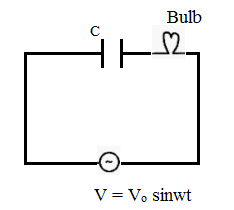There is no current through dielectrics of the capacitor.

By increasing the applied voltage: Capacitor starts charging and starts to flow from one place to another in one direction.

When the applied voltage is decreased: Capacitor starts discharging. Now the direction of charge transfer is reversed.

Capacitor alternatively charges and discharges}→ When an AC voltage is given to it.

Capacitor reactance with AC and DC: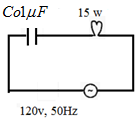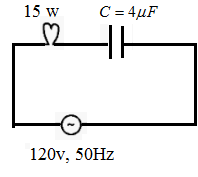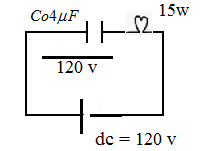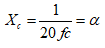A 4μf capacitors connected in For this case Bulb glows less For DC w = 0

Series to the Bulb the Bulb glow Bright then 4μF capacitor for DC capacitor applies ∞

When it is shining brightly, we get i = Vo / Xc = Vo WC resistance whole of potential i α c is dropped across the capacity so the Bulb cannot light up.

Case (II): Series and parallel capacitive reactance

Xc – Capacitive reactance is nothing opposition to the ac current. They behave as Resistors they will be assumed the same as resistors connected in series and parallel.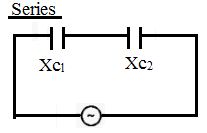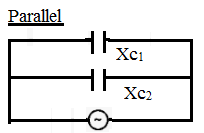Xc1 = Xc1 + Xc2 + … $\frac{1}{X{{c}_{T}}}=\frac{1}{X{{c}_{1}}}+\frac{1}{X{{c}_{2}}}+….$

Case (III): Current through capacitive reactance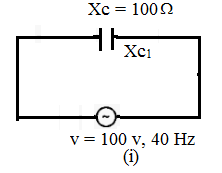$I=\frac{V}{{{X}_{c}}}=1A$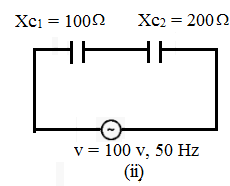${{V}_{1}}+{{V}_{2}}=IX{{c}_{1}}+IX{{c}_{2}}$ $V=I(x{{c}_{1}}+x{{c}_{2}})$ $\frac{v}{{{X}_{ct}}}=I=\frac{1}{3}A$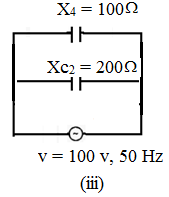$I={{I}_{1}}+{{I}_{2}} \\ \,{{V}_{T}}=\frac{v}{X{{c}_{1}}}+\frac{v}{X{{c}_{2}}}=1A \\$

Application of Capacitive Reactance

Xc – Blocks the DC current

Xc – Offers low reactance to AC.

Solved Problems:

Problem 1: Find out the capacitive reactance (Xc) for an AC source of 100v and 50 Hz by a capacitor of c = 20 μF.
Solution:

${{X}_{c}}=\frac{1}{wc}=\frac{1}{2\pi fc}=\frac{1}{2\pi \times 50\times 20\times {{10}^{-6}}}=\frac{{{10}^{6}}}{1000\pi }=\frac{{{10}^{3}}}{\pi }$ ${{X}_{c}}=\frac{{{10}^{3}}}{\pi }$

Problem 2: A capacitor offers Resistance of 100Ω to an AC source of 100 v and 50 Hw find out the value of the capacitor. C = ?

Solution:

${{X}_{c}}=\frac{1}{wc}\Rightarrow c=\frac{1}{{{x}_{c}}w}=\frac{1}{100\times 2\pi \times 50} \\ {{x}_{c}}=\frac{{{10}^{-4}}}{\pi } \\$

Common Questions

1. What happens to the XC as the frequency of an AC source decreases?

${{X}_{c}}\alpha \frac{1}{f}$

F – decreases, Xc – increases

2. What happens to the XC as the frequency of an AC source increases?

${{X}_{c}}\alpha \frac{1}{f}$

Increases in f 🡪cause Xc to decrease

3. What happens to the Xc for smaller capacitor?

${{X}_{c}}\alpha \frac{1}{c}$ for Higher c, Xc = small